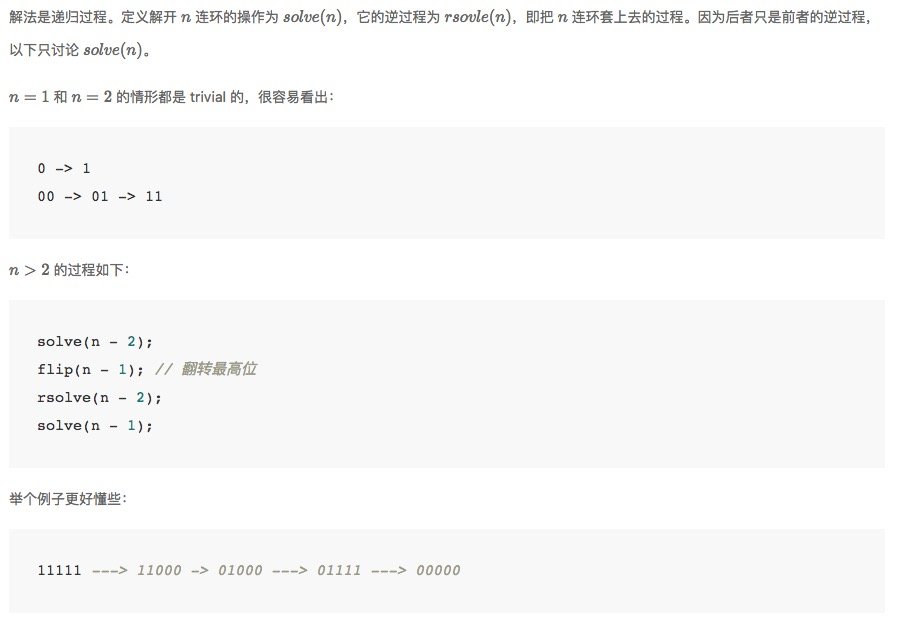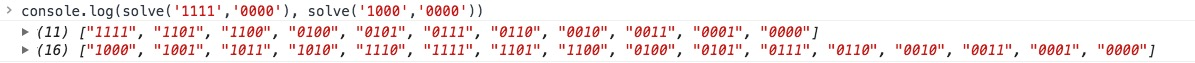## 九连环与格雷码

### 九连环的原理

• 对于最右边的环，可以任意解开或套上
• 对于其他环，只有在「右边环套上，右边的右边全部解开」的情况下，才能解开或套上

### 四连环的所有步骤

0 0000 1111 0000
1 0001 1101 0001
2 0010 1100 0011
3 0011 0100 0010
4 0100 0101 0110
5 0101 0111 0111
6 0110 0110 0101
7 0111 0010 0100
8 1000 0011 1100
9 1001 0001 1101
10 1010 0000 1111

### 程序解法• 活动最右边的环
• 活动右边状态为 1(0...) 的环

• 彻底解开九连环必须解开最里面的环（我们称之为 r0, 其右边的环依次称为 r1/r2/.../r8）
• 要解开 r0，我们期望 r1 状态为 1
• 接下来要分情况讨论，如果 r1 为 1，那么我们期望 r2～r8 状态全都是 0；如果 r1 为 0，我们必须先把它变成 1，而要把 r1 变成 1，我们期望 r2~r8 的状态为 1(0...)
• 以此类推……

``````function nextStepOf (s) {
var a = s.split('').map(v => parseInt(v))
var limit = a.length - 1
expect(0, 0)
return a.join('')
function expect (index, value) { // 期望 a[index] 值为 value
if (index === limit) {
if (a[index] === value) {
a[index - 1] = a[index - 1] ^ 1
} else {
a[index] = value
}
return
}
if (a[index] !== value) {
if (oneWithAllZero(index + 1)) { // 右边状态为 1(0...)
a[index] = value
} else {
expect(index + 1, 1)
}
} else {
expect(index + 1, 0)
}
}
function oneWithAllZero (index) {
if (!a[index]) return false
for (var i = index + 1; i <= limit; i++) {
if (a[i]) {
return false
}
}
return true
}
}

function solve (start, final) {
var result = [start]
while (start !== final) {
start = nextStepOf(start)
result.push(start)
}
return result
}

console.log(solve('1111','0000'), solve('1000','0000'))``````expect 期望值和环的实际状态相符 期望值和环的实际状态不符

### 格雷码的生成方法

``````function generateGrayCode (n) {
var start = Array(n).fill(0)
var flag = true
var result = []
result.push(start.slice())
for (var i = 0; i < (1 << n) - 1; i++) {
if (flag) {
start = changeRightMost(start)
result.push(start.slice())
} else {
start = changeOther(start)
result.push(start.slice())
}
flag = !flag
}
return result
}

function changeRightMost (arr) { // 改变最右边的位元
arr[arr.length - 1] = arr[arr.length - 1] ^ 1
return arr
}

function changeOther (arr) { // 改变右起第一个为1的位元的左边位元
for (var i = arr.length - 1; i > 0; i--) {
if (arr[i]) {
arr[i - 1] = arr[i - 1] ^ 1
break
}
}
return arr
}

console.table(generateGrayCode(4))``````

### 格雷码和二进制数的关系

0 ^ b3 = g3
b3 ^ b2 = g2
b2 ^ b1 = g1
b1 ^ b0' = g0'

``````function grayToBinary (s) {
var r = 
for (var i = 1; i <= s.length; i++) {
r[i] = r[i - 1] ^ s[i - 1]
}
r.shift()
return r.join('')
}
grayToBinary('0111') // '0101'

function binaryToGray (s) {
var r = []
var a = s.split('').map(v => parseInt(v))
a.unshift(0)
for (var i = 0; i < s.length; i++) {
r[i] = a[i] ^ a[i + 1]
}
return r.join('')
}
binaryToGray('0101') // '0111'``````